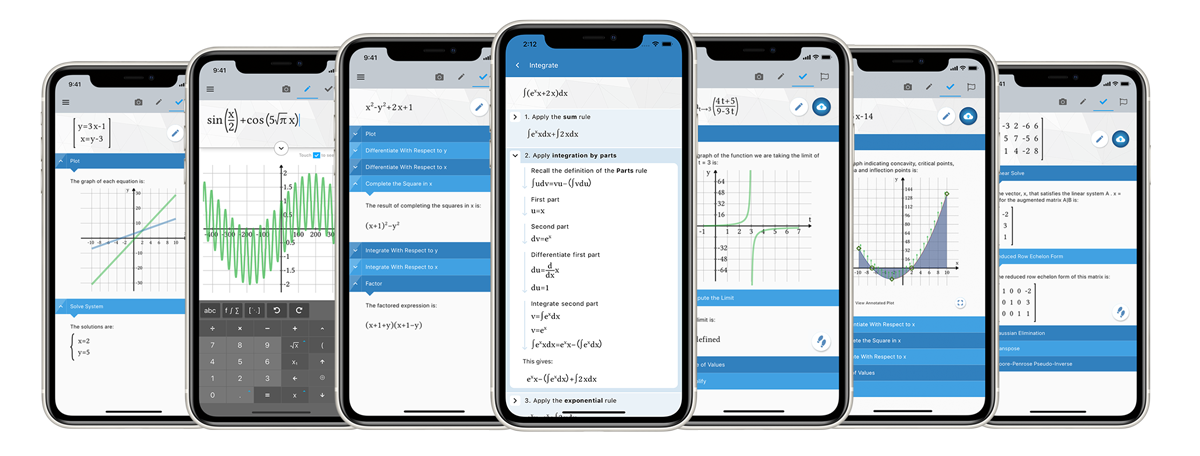Maplesoft Media Releases

# Maplesoft Media Releases

### New release of Maple Calculator app helps students learn math with step-by-step solutions

##### App provides access to the powerful Maple math engine from an intuitive mobile interface

Waterloo, Canada; Mar 2, 2021 Maplesoft today announced a new release of Maple Calculator, a free mobile app that lets students solve math problems, check homework, and explore graphs from a wide variety of mathematical topics. With the app, students can enter, solve, and visualize mathematical problems from algebra, precalculus, calculus, linear algebra, and differential equations. With the latest release, Maple Calculator not only solves the problem, but in most cases, also provides a step-by-step solution so that students can learn how to solve the problem themselves.Students can enter problems into Maple Calculator using the phone’s camera. They can take a picture of a handwritten or typeset math problem, and the app will use AI technology to translate the image. Alternatively, they can enter the problem directly using the same mathematical notation they would use in their own notebooks. Once entered, students can choose to perform a variety of mathematical operations, such as graphing the 2-D curve or 3-D surface, finding an integral, solving a system of equations, inverting a matrix, factoring a polynomial, and much more. The available operations change according to the mathematical expression that was entered, so they are always relevant.

With the new release, students also have the option to then ask for the steps that lead to the given result. Consequently, students can use the app not just to check if their own work is correct, but to find the source of the problem if they made a mistake. Students can also use the steps to learn how to approach problems they are unfamiliar with. Steps are available in Maple Calculator for a wide variety of problems, including solving equations and systems of equations, finding limits, derivatives, and integrals, and performing matrix operations such as finding inverses and eigenvalues.

“Students around the world are using Maple Calculator to check their homework, visualize problems and solutions, experiment with ‘what-if’ scenarios and more, and all these activities help them deepen their mathematical understanding and improve their confidence,” says Karishma Punwani, Director of Academic Product Management at Maplesoft. “Mathematics education is incredibly important, and is especially challenging this year with so many students learning remotely. So we’re especially gratified that, with the addition of step by step solutions in this new release, Maple Calculator will be even more helpful for students learning math.”

Maple Calculator is part of the Maple Math Suite, which provide access to the world’s most powerful mathematics engine through a variety of specialized, easy-to-use interfaces that make it extremely easy to explore, visualize, and solve mathematical problems in different situations. Other products include Maple, the world-leading mathematical software used by researchers, educators, and students around the world, and the recently introduced Maple Learn™, an online environment for teaching, learning, and doing math from high school to the second year of university. Maple Calculator is complementary to both products, and can be used to avoid transcription errors when entering mathematical expressions in Maple or Maple Learn. By using Maple Calculator to take a picture of their math, users can easily upload those mathematical expressions to Maple or Maple Learn for further explorations.

The free Maple Calculator app is available from the Apple App Store for iOS, and from Google Play for Android users. For more information about Maple Calculator, visit https://www.maplesoft.com/products/MapleCalculator.